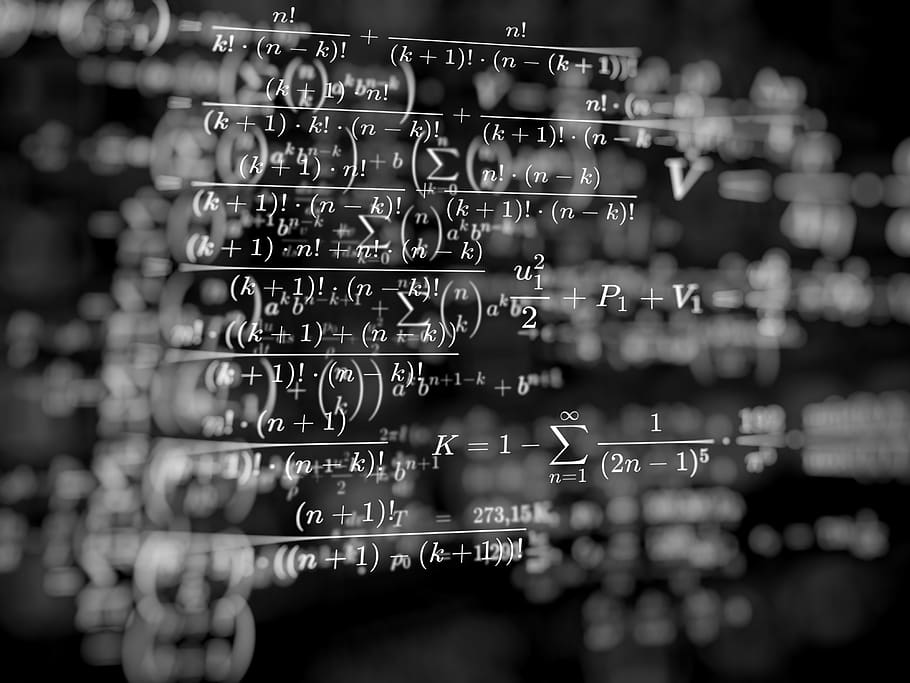Tehran Institute for Advanced Studies (TeIAS)

# Mathematics of Data ScienceThis course covers a wide range of mathematical tools that are helpful in data science. The course mostly focuses on Linear Algebra, Probability, Random Graphs and Statistics.

## Learning Objectives

The linear algebra part covers concepts like vector space, inner product, change of basis, elementary row/column operations, rank, eigenvalues and eigenvectors, linear operators, Matrix representation, functions of operators, adjoint, Schur decomposition, Unitary, Hermitian, normal, singular value decomposition as well as Direct sum and tensor product of vector spaces.

The course then proceeds with basic concepts in probability such as law of large numbers, concentration of measure as well as topics in random graph theory. Basic concepts in statistics such as hypothesis testing are also reviewed.

## Announcements

(No information at the moment, a place holder for future announcements.)